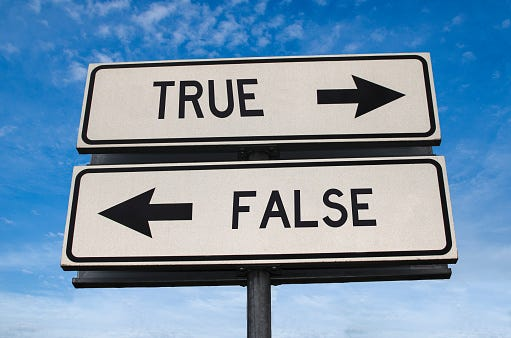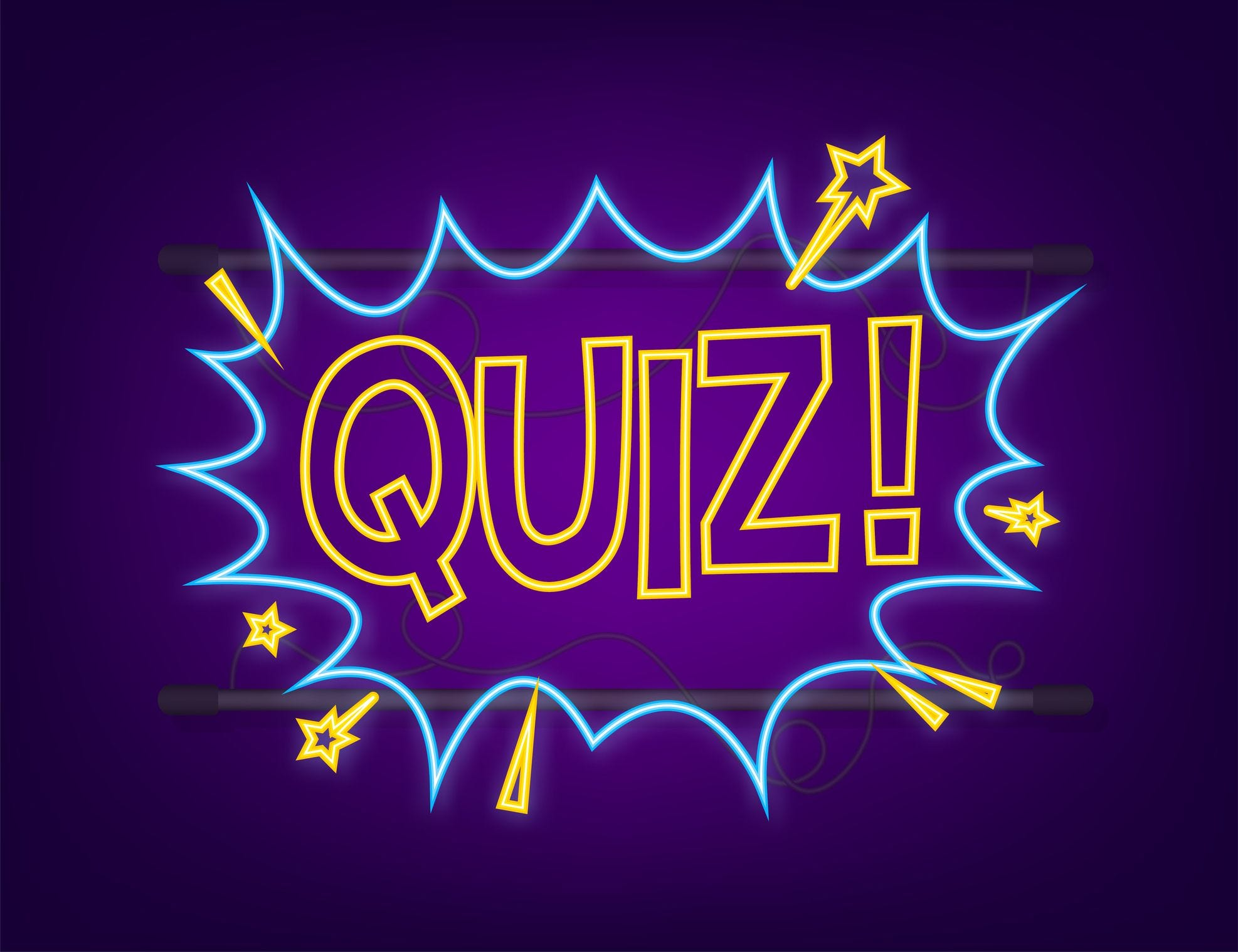# JavaScript Quiz #06 - Truthy and Falsy Values

What are Truthy and Falsy Values In JavaScript? How to use Truthy and Falsy Values in JavaScript?In JavaScript, values can be classified as either truthy or falsy. Knowing which values are considered truthy or falsy allows you to write more concise and effective code. Let’s explore truthy and falsy values in JavaScript with code examples.

## Truthy Values:

Truthy value is any value that is considered true when evaluated in a Boolean context. The following values are considered truthy:

1. Non-empty strings: Any string that contains at least one character is considered truthy.

``````    var name = "John";
if (name) {
console.log("Hello, " + name);
}

Output: "Hello, John"
``````

2. Numbers: Any nonzero number (positive or negative) is considered truthy.

``````    var count = 42;
if (count) {
console.log("Count is: " + count);
}

Output: "Count is: 42"
``````

3. Objects: Any JavaScript object, including arrays and functions, is considered truthy.

``````    var person = {
name: "Alice",
age: 25
};
if (person) {
console.log("Person's name is: " + person.name);
}

Output: "Person's name is: Alice"
``````

4. Arrays: Even an empty array is considered truthy.

``````    var myArray = [];
if (myArray) {
console.log("Array is not empty");
}

Output: "Array is not empty"
``````

## Falsy Values:

A falsy value is any value that is considered false when evaluated in a Boolean context. The following values are considered falsy:

1. Empty strings: An empty string, represented by “ ”, is considered falsy.

``````    var username = "";
}

``````

2. Zero: The number zero (0) is considered falsy.

``````    var quantity = 0;
if (!quantity) {
console.log("No items in the cart");
}

Output: "No items in the cart"
``````

3. NaN: The special value NaN (Not a Number) is considered falsy.

``````    var result = parseInt("abc");
if (!result) {
console.log("Invalid number");
}

Output: "Invalid number"
``````

4. null and undefined: Both null and undefined are considered falsy.

``````    var data = null;
if (!data) {
console.log("No data available");
}

Output: "No data available"
``````

## Quizzes• What is the output?
``````console.log(!!"0");
``````

a) true

b) false

c) 0

The code snippet uses the double negation (!!) operator, which coerces the value to its corresponding boolean representation. In JavaScript, any non-empty string is considered truthy. Therefore, “0” is coerced to true, and the result is true.

2. What is the output?

``````var x = " ";
console.log(Boolean(x));
``````

a) true

b) false

c) “ “

The Boolean() function is used to explicitly convert a value to its boolean representation. In JavaScript, an empty string is considered falsy, but a string with a single space character (“ “) is considered truthy. Therefore, Boolean(x) evaluates to true, and the result is true.

3. What is the output?

``````console.log(Boolean(Symbol()));
``````

a) true

b) false

c) Symbol

A JavaScript Symbol is considered a truthy value. Therefore, Boolean(Symbol()) evaluates to true, and the result is true.

4. Which of the following values is considered falsy in JavaScript?

a) 0

b)

c) []

d) -1

0 is considered falsy, while objects (including empty objects ) and arrays (including empty arrays []) are considered truthy. The number -1 is also truthy.

5. What is the output?

``````console.log(0 || "Hello" && (null ?? true));
``````

a) 0

b) “Hello”

c) null

d) true

0 || "Hello" evaluates to "Hello" because 0 is falsy and the OR operator returns the first truthy value encountered."Hello" && null evaluates to null because the AND operator returns the last falsy value encountered.null ?? true evaluates to true because null is considered falsy, and the nullish coalescing operator provides the default value true.

Therefore, the result is true.

6. What is the output?

``````console.log("" === false);
``````

a) true

b) false

The strict equality operator (===) compares the value and the type of the operands. In this case, an empty string ("") is a falsy value, while false is a boolean value. Since they have different types, the comparison evaluates to false.

7. What is the output?

``````console.log(Boolean(-42));
``````

a) true?

b) false

c) 0

any nonzero number is considered truthy, including the negative number -42. Therefore, Boolean(-42) evaluates to true, and the result is true

8. What is the output?

``````let obj = {
prop1: {
prop2: null
}
};

console.log(obj.prop1?.prop2 ?? "Default value");
``````

a) null

b) “Default value”

c) false

The code snippet uses optional chaining (?.) to check if obj.prop1.prop2 is defined. If it is not defined or evaluates to null or undefined, the nullish coalescing operator (??) provides a default value of "Default value". In this case, obj.prop1.prop2 is null, so the result is "Default value".

9. What is the output?

`````` console.log(Boolean(-0))
``````

a) true

b) false

c) 0

Ans: false

Both positive zero (+0) and negative zero(-0) are considered falsy.

https://developer.mozilla.org/en-US/docs/Glossary/Falsy